GMAT Math : DSQ: Calculating arithmetic mean

Example Questions

Example Question #1178 : Data Sufficiency Questions

Choose the answer that best describes sufficient data to solve the problem.

3 numbers are given in increasing order.  The arithemetic mean of the first two is 5 less than the arithmetic mean of all three.  The sum of the first two numbers is equal to the arithmetic mean of the last two. What is the first number?

I. The second number is given.

II. The arithmetic mean of the first and third numbers is given.

Either statement alone is sufficient to solve the problem.

Statement I is sufficient to solve the problem, but Statement II alone is not.

Both statements together are sufficient to solve the problem.

Neither statement is sufficient to solve the problem (additional information is needed).

Statement II is sufficient to solve the problem, but Statement I alone is not.

Statement I is sufficient to solve the problem, but Statement II alone is not.

Explanation:

3 numbers are given in increasing order.I. The second number is given.is determined

II. The arithmetic mean of the first and third numbers is given.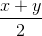is given, and therefore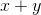is given.

We are given a system of equations by the prompt:

The arithemetic mean of the first two is 5 less than the arithmetic mean of all three.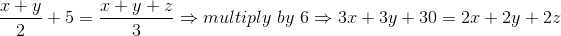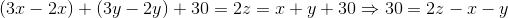The sum of the first two numbers is equal to the arithmetic mean of the last two.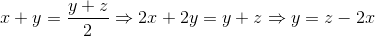Combining these two, we get: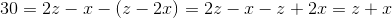Thus, the second statement doesn't actually provide us with any information (we are still left with 2 equations and 3 variables, which cannot be solved for any particular number).

On the other hand, if y is determined, then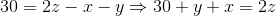relatesand.  Similarly,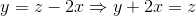relatesand.  Since these give different equations, we could use them to solve for bothand.

So the first gives sufficient data while the second does not.

Example Question #1179 : Data Sufficiency Questions

1. If the arithmetic mean of five different numbers is 50, how many of the numbers are greater than 50?

(1) None of the five numbers is greater than 100.

(2) Three of the five numbers are 24, 25 and 26, respectively.

BOTH statements TOGETHER are sufficient, but NEITHER statement ALONE is sufficient.

EACH statement ALONE is sufficient.

Statement (1) ALONE is sufficient, but statement (2) alone is not sufficient.

Statement (2) ALONE is sufficient, but statement (1) alone is not sufficient.

Statements (1) and (2) TOGETHER are NOT sufficient.

BOTH statements TOGETHER are sufficient, but NEITHER statement ALONE is sufficient.

Explanation:

For statement (1), there are different combinations that satisfy the condition. For example, the five numbers can be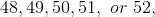or the five numbers can be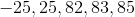. Therefore, we cannot determine how many of the numbers are greater thanby knowing the first statement.

For statement (2), even though we know three of them, the two unknown numbers can both be greater than, or one smaller and one greater. Thus statement (2) is not sufficient.

Putting the two statements together, we know that the sum of the two unknown numbers is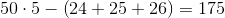Since none of them is greater than 100, both of them have to be greater than 50. Therefore when we combine the two statements, we know that there are two numbers that are greater than 50.

Example Question #1180 : Data Sufficiency Questions

Data sufficiency question

Determine the mean of a number set.

1. The mode is 7.

2. The median is 7.

Each statement alone is sufficient

Statement 2 alone is sufficient, but statement 1 alone is not sufficient to answer the question

Statements 1 and 2 together are not sufficient, and additional data is needed to answer the question

Statement 1 alone is sufficient, but statement 2 alone is not sufficient to answer the question

Both statements taken together are sufficient to answer the question, but neither statement alone is sufficient

Statements 1 and 2 together are not sufficient, and additional data is needed to answer the question

Explanation:

The median gives information about the center of a set of numbers, but is insufficient for calculating the mean. Additionally, the mode merely indicates which number is the most repeated value. Therefore, more information is required to calculate the mean.

Example Question #31 : Descriptive Statistics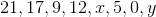What is the value of?

(1)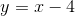(2) The arithmetic mean of the numbers in the list is.

Both statements TOGETHER are sufficient, but NEITHER statement ALONE is sufficient.

Statement (2) ALONE is sufficient, but Statement (1) ALONE is not sufficient.

Both statements TOGETHER are not sufficient.

Statement (1) ALONE is sufficient, but Statement (2) ALONE is not sufficient.

Each statement ALONE is sufficient.

Both statements TOGETHER are sufficient, but NEITHER statement ALONE is sufficient.

Explanation:

Statement (1) provides the value of y in terms of x, which is not enough to determine the value of x in the list we are given.

Statement (2) gives the arithmetic mean of the list. We can the write the following equation: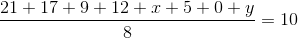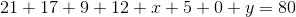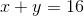However, we cannot find the value of x using the information in Statement (2) only.

Using the information in Statement (1), we can replace y by x-4 in the previous equation: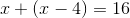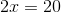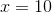We need both statements to calculate the value of x.

Both statements TOGETHER are sufficient, but NEITHER statement ALONE is sufficient.

Example Question #32 : Descriptive Statistics

A professor records the average class grade for each exam. The average class grades for the semester are respectively: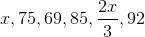What is the average class grade for the semester?

(1)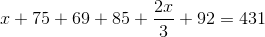(2)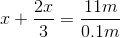Statement (1) ALONE is sufficient, but Statement (2) ALONE is not sufficient

Both statements TOGETHER are not sufficient.

Both statements TOGETHER are sufficient, but NEITHER statement ALONE is sufficient

Each statement ALONE is SUFFICIENT

Statement (2) ALONE is sufficient, but Statement (1) ALONE is not sufficient

Each statement ALONE is SUFFICIENT

Explanation:

The mean class grade is the sum of all average class grades divided by the number of grades: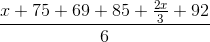Using Statement (1):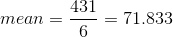Therefore Statement (1) is sufficient to calculate the arithmetic mean for these grades.

Using statement (2):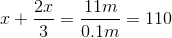Therefore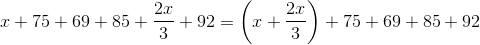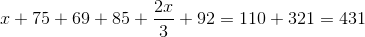Therefore Statement (2) is sufficient to calculate the arithmetic mean for these grades.

Each Statement ALONE IS SUFFICIENT to answer the question

Example Question #33 : Descriptive Statistics

During a particularly hectic week at Ballard High, Robert drank 5, 8, 3, 6, 2, 9, and 14 cans of Slurp Soda, respectively,  on each of the 7 days. What is the product of Robert's mean and median soda consumption for that week?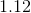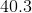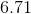Explanation:

To find the mean, we find the sum of all of the values, and divide by how many there are: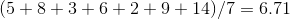To find the mean we rearrange the values in ascending numerical order and select the middle value: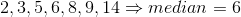The product, then, is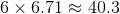Example Question #34 : Descriptive Statistics

Give the arithmetic mean ofand.

Statement 1: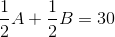Statement 2: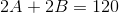BOTH STATEMENTS TOGETHER do NOT provide sufficient information to answer the question.

BOTH STATEMENTS TOGETHER provide sufficient information to answer the question, but NEITHER STATEMENT ALONE provides sufficient information to answer the question.

STATEMENT 1 ALONE provides sufficient information to answer the question, but STATEMENT 2 ALONE does NOT provide sufficient information to answer the question.

EITHER STATEMENT ALONE provides sufficient information to answer the question.

STATEMENT 2 ALONE provides sufficient information to answer the question, but STATEMENT 1 ALONE does NOT provide sufficient information to answer the question.

EITHER STATEMENT ALONE provides sufficient information to answer the question.

Explanation:

The arithmetic mean ofandis equal to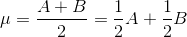.

Statement 1 alone gives us this value directly.

From Statement 2 alone, the value can be determined by dividing both sides by 4: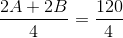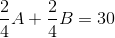Example Question #35 : Descriptive Statistics

Given five distinct positive integers -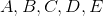- which of them is the median?

Statement 1: The median of, andis.

Statement 2: The median of, and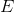is.

BOTH STATEMENTS TOGETHER provide sufficient information to answer the question, but NEITHER STATEMENT ALONE provides sufficient information to answer the question.

STATEMENT 2 ALONE provides sufficient information to answer the question, but STATEMENT 1 ALONE does NOT provide sufficient information to answer the question.

EITHER STATEMENT ALONE provides sufficient information to answer the question.

STATEMENT 1 ALONE provides sufficient information to answer the question, but STATEMENT 2 ALONE does NOT provide sufficient information to answer the question.

BOTH STATEMENTS TOGETHER do NOT provide sufficient information to answer the question.

BOTH STATEMENTS TOGETHER provide sufficient information to answer the question, but NEITHER STATEMENT ALONE provides sufficient information to answer the question.

Explanation:

The median of three or five numbers (both odd numbers) is the number in the middle when they are arranged in ascending order. Statement 1 alone does not answer the question of which one is the median; both of the following orderings are consistent with that statement: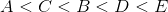- median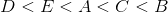- median.

For similar reasons, Statement 2 alone does not answer the question.

Now, assume both statements to be true. From Statement 1, exactly one ofandis less than, and the other is greater. From Statement 2, exactly one ofandis less than, and the other is greater. Therefore, two of the five values are less thanand two are greater, makingthe middle element, or median.

Example Question #36 : Descriptive Statistics

Give the arithmetic mean of the second and third terms of an arithmetic sequence.

Statement 1: The fourth term of the sequence is 120.

Statement 2: The first term of the sequence is 0.

BOTH STATEMENTS TOGETHER provide sufficient information to answer the question, but NEITHER STATEMENT ALONE provides sufficient information to answer the question.

STATEMENT 2 ALONE provides sufficient information to answer the question, but STATEMENT 1 ALONE does NOT provide sufficient information to answer the question.

BOTH STATEMENTS TOGETHER do NOT provide sufficient information to answer the question.

EITHER STATEMENT ALONE provides sufficient information to answer the question.

STATEMENT 1 ALONE provides sufficient information to answer the question, but STATEMENT 2 ALONE does NOT provide sufficient information to answer the question.

BOTH STATEMENTS TOGETHER provide sufficient information to answer the question, but NEITHER STATEMENT ALONE provides sufficient information to answer the question.

Explanation:

Assume Statement 1 alone. The sequences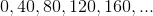and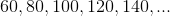are both arithmetic, each term being the previous term plus the same number (in the first case, this common difference is 40; in the second, it is 20). The fourth term is 120 in both cases, The second and third terms of the first sequence have arithmetic mean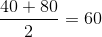; the second and third terms of the second sequence have arithmetic mean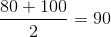.  Therefore, the mean of those two terms cannot be determined for certain. A similar argument holds for Statement 2 alone being insufficient.

Now assume both statements. Letbe the common difference of the sequences mentioned in Statement 2. By Statement 2, 0 is the first term, so the sequence will be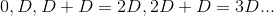By the first statement, the fourth term is 120, so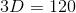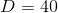The second terms isand the third term is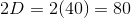, and their arithmetic mean is.

Example Question #37 : Descriptive Statistics

Give the arithmetic mean of the first and third terms of an arithmetic sequence.

Statement 1: The fifth term of the sequence is 130.

Statement 2: The second term of the sequence is 100.

EITHER STATEMENT ALONE provides sufficient information to answer the question.

BOTH STATEMENTS TOGETHER provide sufficient information to answer the question, but NEITHER STATEMENT ALONE provides sufficient information to answer the question.

BOTH STATEMENTS TOGETHER do NOT provide sufficient information to answer the question.

STATEMENT 2 ALONE provides sufficient information to answer the question, but STATEMENT 1 ALONE does NOT provide sufficient information to answer the question.

STATEMENT 1 ALONE provides sufficient information to answer the question, but STATEMENT 2 ALONE does NOT provide sufficient information to answer the question.

STATEMENT 2 ALONE provides sufficient information to answer the question, but STATEMENT 1 ALONE does NOT provide sufficient information to answer the question.

Explanation:

An arithmetic sequence is one in which each term is formed by adding the same number to its preceding term - the common difference.

Letbe the first term, andbe the common difference. The first five terms are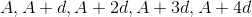The arithmetic mean of two numbers is half the sum of the numbers. The arithmetic mean of the first and third terms is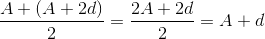,

which is the second term. Statement 2 alone gives this number as 100.

Now assume Statement 1 alone. Consider these two sequences, both of which can be seen to be arithmetic with fifth term 130: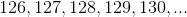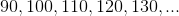The arithmetic mean of the first and third terms differ, as can be seen by looking at the second terms; in the first sequence, it is 127, and in the second, it is 100. That makes Statement 2 inconclusive.

Tired of practice problems?

Try live online GMAT prep today.